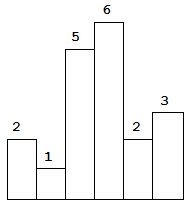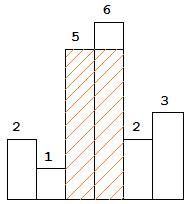# LeetCode 84. Largest Rectangle in Histogram

## Description

Largest Rectangle in Histogram

Given n non-negative integers representing the histogram’s bar height where the width of each bar is 1, find the area of largest rectangle in the histogram.Above is a histogram where width of each bar is `1`, given height = `[2,1,5,6,2,3]`.The largest rectangle is shown in the shaded area, which has area = `10` unit.

# LeetCode 82. Remove Duplicates From Sorted List II

## Description

Remove Duplicates from Sorted List II

Given a sorted linked list, delete all nodes that have duplicate numbers, leaving only distinct numbers from the original list.

Example 1:

``````Input: 1->2->3->3->4->4->5
Output: 1->2->5
``````

Example 2:

``````Input: 1->1->1->2->3
Output: 2->3
``````

# LeetCode 81. Search in Rotated Sorted Array II

## Description

Search in Rotated Sorted Array II

Suppose an array sorted in ascending order is rotated at some pivot unknown to you beforehand.

(i.e., `[0,0,1,2,2,5,6]` might become `[2,5,6,0,0,1,2]`).

You are given a target value to search. If found in the array return `true`, otherwise return `false`.

Example 1:

``````Input: nums = [2,5,6,0,0,1,2], target = 0
Output: true
``````

Example 2:

``````Input: nums = [2,5,6,0,0,1,2], target = 3
Output: false
``````

• This is a follow up problem to Search in Rotated Sorted Array, where nums may contain duplicates.
• Would this affect the run-time complexity? How and why?

# LeetCode 80. Remove Duplicates from Sorted Array II

## Description

Remove Duplicates from Sorted Array II

Given a sorted array nums, remove the duplicates in-place such that duplicates appeared at most twice and return the new length.

Do not allocate extra space for another array, you must do this by modifying the input array in-place with O(1) extra memory.

Example 1:

``````Given nums = [1,1,1,2,2,3],

Your function should return length = 5, with the first five elements
of nums being 1, 1, 2, 2 and 3 respectively.

It doesn't matter what you leave beyond the returned length.
``````

Example 2:

``````Given nums = [0,0,1,1,1,1,2,3,3],

Your function should return length = 7, with the first seven elements
of nums being modified to 0, 0, 1, 1, 2, 3 and 3 respectively.

It doesn't matter what values are set beyond the returned length.
``````

# LeetCode 79. Word Search

## Description

Word Search

Given a 2D board and a word, find if the word exists in the grid.

The word can be constructed from letters of sequentially adjacent cell, where “adjacent” cells are those horizontally or vertically neighboring. The same letter cell may not be used more than once.

Example:

``````board =
[
['A','B','C','E'],
['S','F','C','S'],
['A','D','E','E']
]

Given word = "ABCCED", return true.
Given word = "SEE", return true.
Given word = "ABCB", return false.
``````

`board`中查找是否存在相连（相邻）的字符与给定的单词对应。

# LeetCode 78. Subsets

## Description

Subsets

Given a set of distinct integers, nums, return all possible subsets (the power set).

Note: The solution set must not contain duplicate subsets.

Example:

``````Input: nums = [1,2,3]
Output:
[
,
,
,
[1,2,3],
[1,3],
[2,3],
[1,2],
[]
]
``````

# LeetCode 77. Combinations

## Description

Combinations

Given two integers n and k, return all possible combinations of k numbers out of 1 … n.

Example:

``````Input: n = 4, k = 2
Output:
[
[2,4],
[3,4],
[2,3],
[1,2],
[1,3],
[1,4],
]
``````

# LeetCode 76. Minimum Window Substring

## Description

Minimum Window Substring

Given a string S and a string T, find the minimum window in S which will contain all the characters in T in complexity O(n).

Example:

``````Input: S = "ADOBECODEBANC", T = "ABC"
Output: "BANC"
``````

Note:

• If there is no such window in S that covers all characters in T, return the empty string “”.
• If there is such window, you are guaranteed that there will always be only one unique minimum window in S.Programer

ChengDu·China